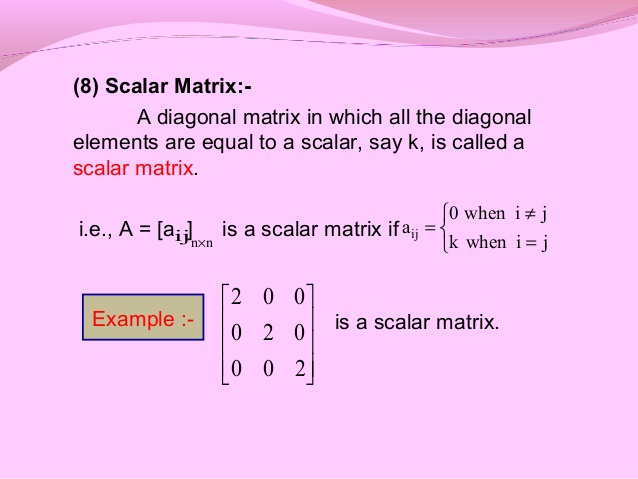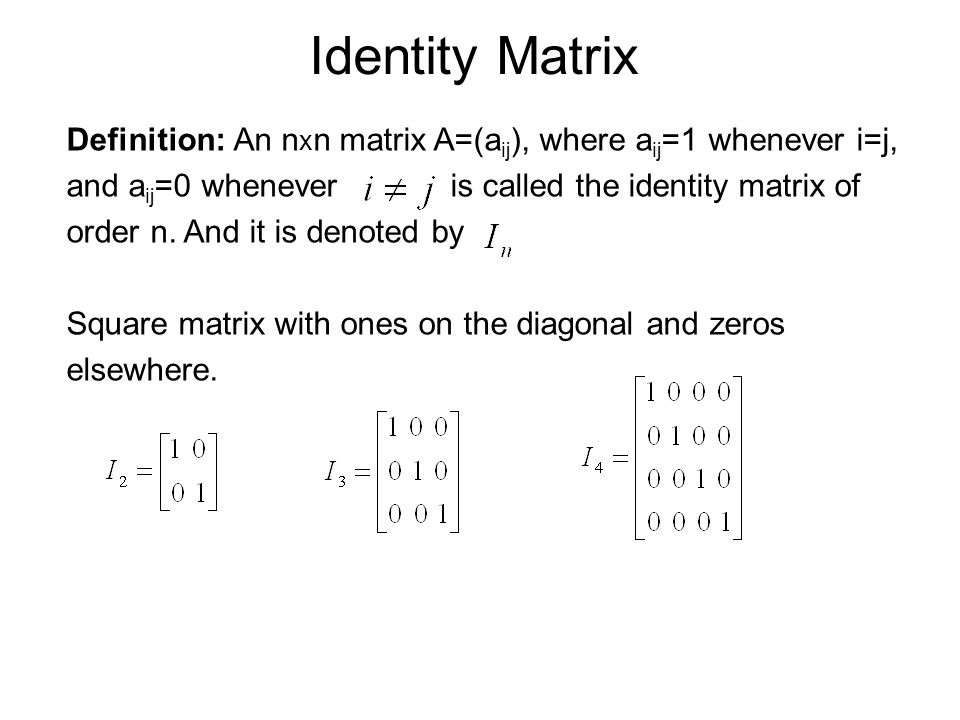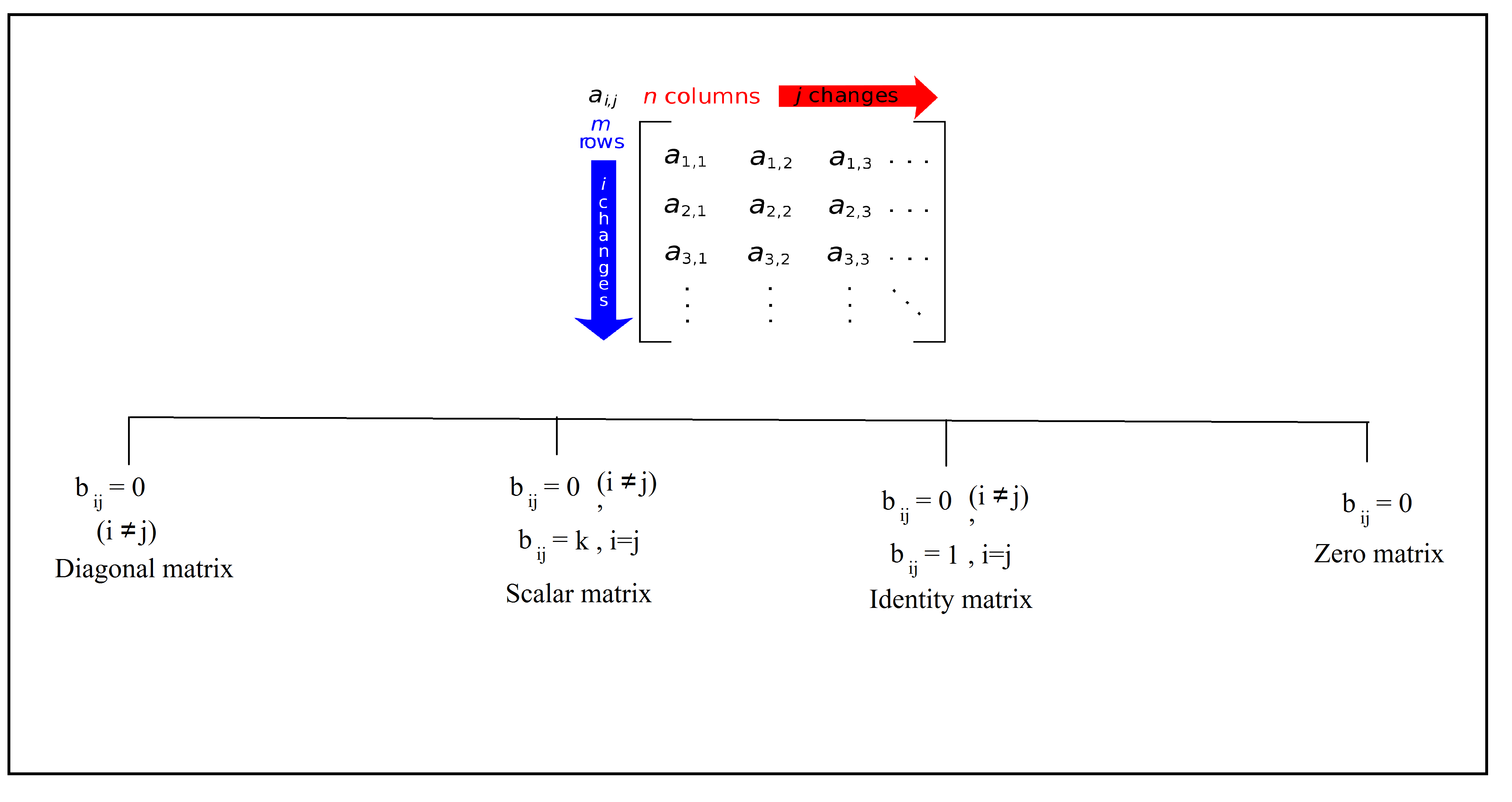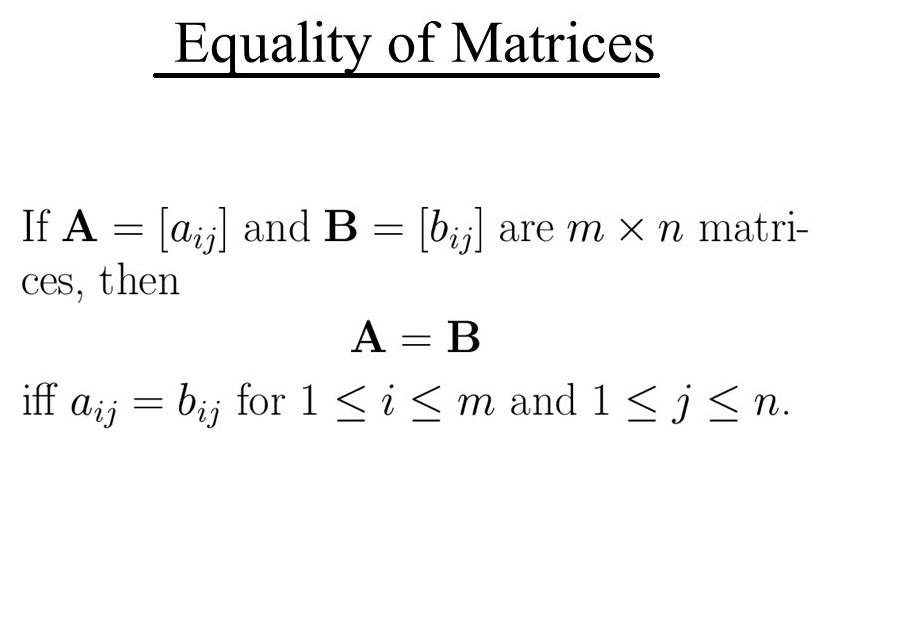Mathematics Matrix For CBSE-NCERT 2

### Topic covered

star Types Of Matrix
star Equality Of Matrix

### Types of matrix

● Diagonal matrix
● Scalar matrix
● Identity matrix
● Zero matrix

### Diagonal matrixcolor{green} ✍️ A square matrix B = [b_(ij)]_(m xx m) is said to be a diagonal matrix if color{green}{"all its non diagonal elements are zero",

color{green} ✍️ A matrix B = [b_(ij)] m xx m is said to be a diagonal matrix color{red } {if b_(ij) = 0, "when" \ \ i ≠ j

E.g. A = , B = [(-1,0),(0,-1)], c = [(-1,0,0),(0,1,0),(0,0,1)]

### Scalar matrixcolor{green} ✍️ A diagonal matrix B = [b_(ij)]_(m xx m) is said to be a scalar matrix if all its color{green}{"non diagonal elements are zero,"}

color{green} ✍️ A matrix B = [b_(ij)] m xx m is said to be a scalar matrix if

color{orange}{
b_(ij) = 0,\ \ "when" \ \ i ≠ j}

color{orange}{
b_(ij) = k "when" \ \ i=j}

E.g. A = , B = [(-1,0),(0,-1)], c = [(5,0,0),(0,5,0),(0,0,5)]

### Identity matrixcolor{green} ✍️ A square matrix in which elements in the diagonal are all 1 and rest are all zero is called an identity matrix. In other words, the square matrix

color{orange}{b_(ij) = 0, \ \ "when" \ \ i ≠ j}
color{orange}{b_(ij) = 1, \ \ "when" \ \ i=j}

E.g. A = , B = [(1,0),(0,1)], c = [(1,0,0),(0,1,0),(0,0,11)]

### Zero matrix

color{green} ✍️ A matrix is said to be zero matrix or null matrix if all its elements are zero.

E.g. A = , B = [(0,0),(0,0)], c = [(0,0,0),(0,0,0),(0,0,0)]

### Summery### Equality of matrices\color{green} ✍️ Two matrices A = [a_(ij)] and B = [b_(ij)] are said to be equal if

(i) they are of the same order
(ii) each element of A is equal to the corresponding element of B, that is a_(ij) = b_(ij) for all i and j.

color{blue}{A = B =>[a_(ij)] = [b_(ij)]}

if [(x,y),(z,a),(b,c)] = [(-1,0),(2,sqrt6),(3,2)]  then x = -, y =0, z=2 ,a = sqrt6, b=3,c=2
Q 3124578451If  [ (x+3, z+4, 2y-7 ), (-6,a-1,0 ), ( b-3, -21 ,0 ) ] = [ (0, 6, 3y-2 ), ( -6, -3, 2c+2), ( 2b+4, -21 , 0 ) ]

Find the values of a, b, c, x, y and z.
Class 12 Chapter 3 Example 4Solution:

As the given matrices are equal, therefore, their corresponding elements
must be equal. Comparing the corresponding elements, we get

x + 3 = 0, z + 4 = 6, 2y – 7 = 3y – 2
a – 1 = – 3, 0 = 2c + 2, b – 3 = 2b + 4,

Simplifying, we get

a = – 2, b = – 7, c = – 1, x = – 3, y = –5, z = 2
Q 3144578453Find the values of a, b, c, and d from the following equation:

[ (2a+b , a-2b ), ( 5c-d, 4c +3d) ] = [ (4,-3), ( 11,24 ) ]
Class 12 Chapter 3 Example 5Solution:

By equality of two matrices, equating the corresponding elements, we get
2a + b = 4, 5c – d = 11
a – 2b = – 3 , 4c + 3d = 24

Solving these equations, we get
a = 1, b = 2, c = 3 and d = 4